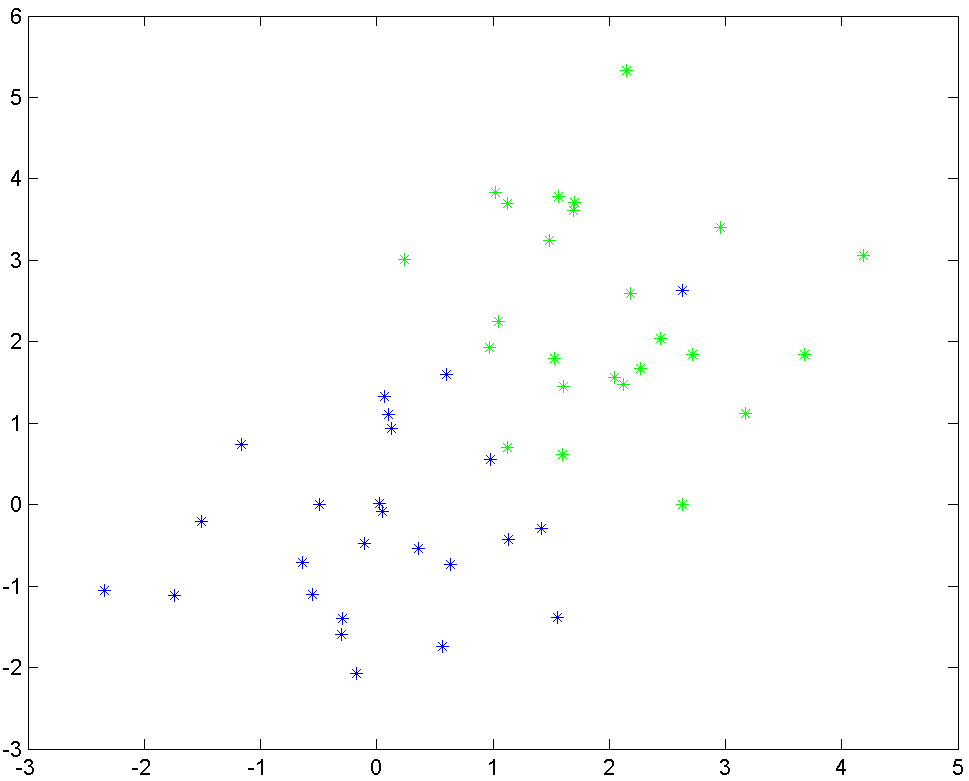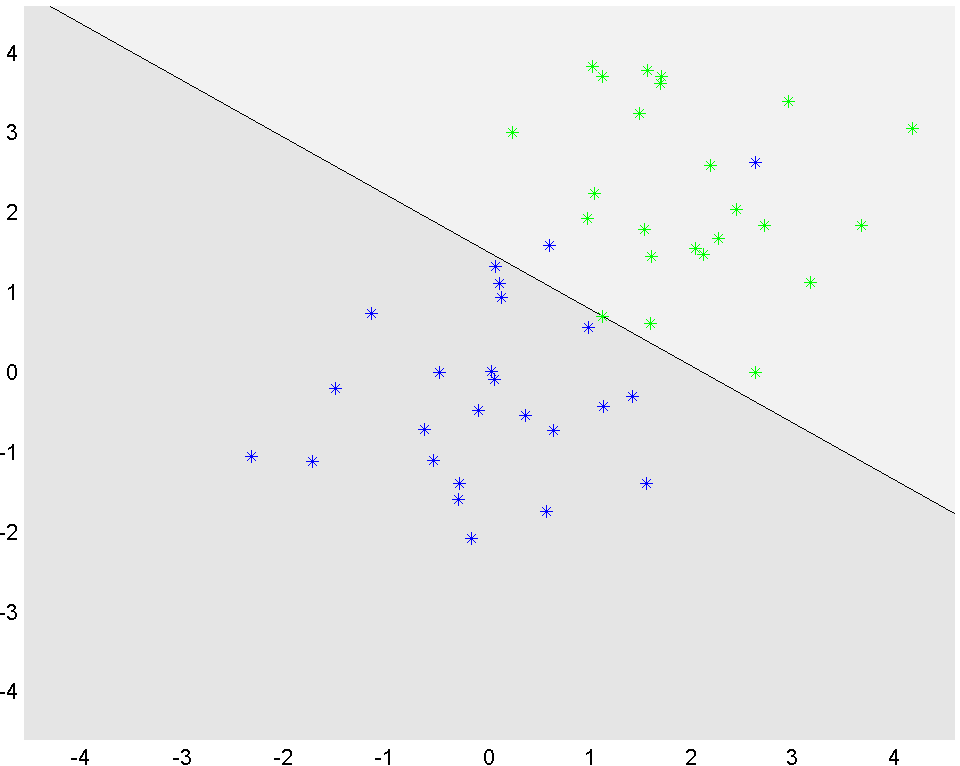# Linear programming

Tags:

Updated:

Complete code, click to expand!

In this example, we are given two sets of data, called blues and greens. Our goal is to separate these sets using a linear classifier.

blues = randn(2,25);
greens = randn(2,25)+2;


Display the two clusters of data.

plot(greens(1,:),greens(2,:),'g*')
hold on
plot(blues(1,:),blues(2,:),'b*')A linear classifier means we want to find a vector $$a$$ and scalar $$b$$ such that $$a^Tx + b \geq 0$$ for all the green points, and $$a^Tx+b\leq 0$$ for all blue points (a separating hyperplane). By looking at the data, it should be clear that this is impossible. What one then would like to do, is to find a hyperplane which misclassifies as few points as possible. This is typically a very hard combinatorial problem, so we will work with an approximation instead.

As a proxy for misclassification, we introduce positive numbers $$u$$ and $$v$$ and change the classification to $$a^Tx+b\geq 1-u$$ and $$a^Tx+b\leq -(1-v)$$. If both $$u$$ and $$v$$ are small, we should obtain a good separation.

We define the decision variables of interest

a = sdpvar(2,1);
b = sdpvar(1);


We will use one $$u$$ and $$v$$ variable for each point, hence we create two vectors of suitable length. It will be obvious below why we define them as row-vectors.

u = sdpvar(1,25);
v = sdpvar(1,25);


The classification constraints are easily defined by exploiting MATLABs and YALMIPs ability to add scalars and vectors

Constraints = [a'*greens+b >= 1-u, a'*blues+b <= -(1-v), u >= 0, v >= 0]


We want $$u$$ and $$v$$ to be small, in some sense, as that indicates a good classification. A simple choice is to minimize the sum of all elements $$u$$ and $$v$$. However, the problem is ill-conditioned in that form, so we add the constraint that the absolute value of all elements in $$a$$ are smaller than $$1$$.

Objective = sum(u)+sum(v)
Constraints = [Constraints, -1 <= a <= 1];


Absolute value is convex and LP-representable, so we could just as well have used the built-in command abs, but here we build the LP as basic as possible.

At last, we are ready to solve the problem

optimize(Constraints,Objective)


The values of the optimal $$a$$ and $$b$$ are obtained using value. To better illustrate the results, we use YALMIPs ability to plot constraint sets to lazily display the separating hyperplane.

x = sdpvar(2,1);
P1 = [-5<=x<=5, value(a)'*x+value(b)>=0];
P2 = [-5<=x<=5, value(a)'*x+value(b)<=0];
clf
plot(P1);hold on
plot(P2);
plot(greens(1,:),greens(2,:),'g*')
plot(blues(1,:),blues(2,:),'b*')